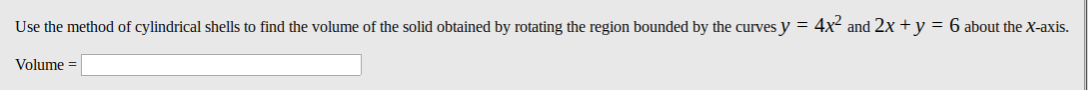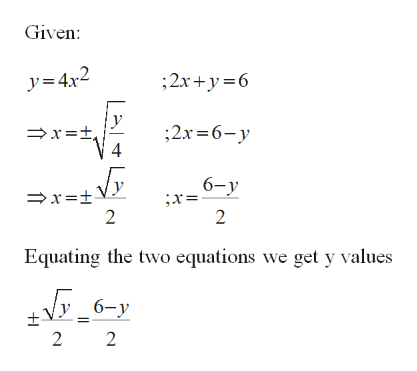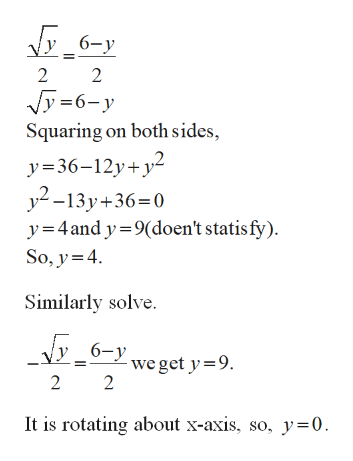# Use the method of cylindrical shells to find the volume of the solid obtained by rotating the region bounded by the curves y = 4x² and 2x+y = 6 about the X-axis.Volume =

Question
10 viewshelp_outlineImage TranscriptioncloseUse the method of cylindrical shells to find the volume of the solid obtained by rotating the region bounded by the curves y = 4x² and 2x+y = 6 about the X-axis. Volume = fullscreen
check_circle

Step 1

Find the volume rotating the region curve and line about x-axis.

If the rotation is about x-axis, then the cylinder is horizontal.

Step 2

Substitute in terms of x, in curve and the line.help_outlineImage TranscriptioncloseGiven: y=4x2 ;2x+y=6 =x=±, 4 ;2x =6-y 6-y ;x= →x=±Vy 2 2 Equating the two equations we get y values +V _ 6-y 2 2. fullscreen
Step 3

Take positive value an...help_outlineImage Transcriptionclosey 6-y 2 Jy =6-y Squaring on both sides, y=36–12y+y² y2 -13y+36=0 y=4and y=9(doen't statis fy). So, y=4. Similarly solve. Jy _6-y - we get y=9. It is rotating about x-axis, so, y=0. fullscreen

### Want to see the full answer?

See Solution

#### Want to see this answer and more?

Solutions are written by subject experts who are available 24/7. Questions are typically answered within 1 hour.*

See Solution
*Response times may vary by subject and question.
Tagged in

### Other